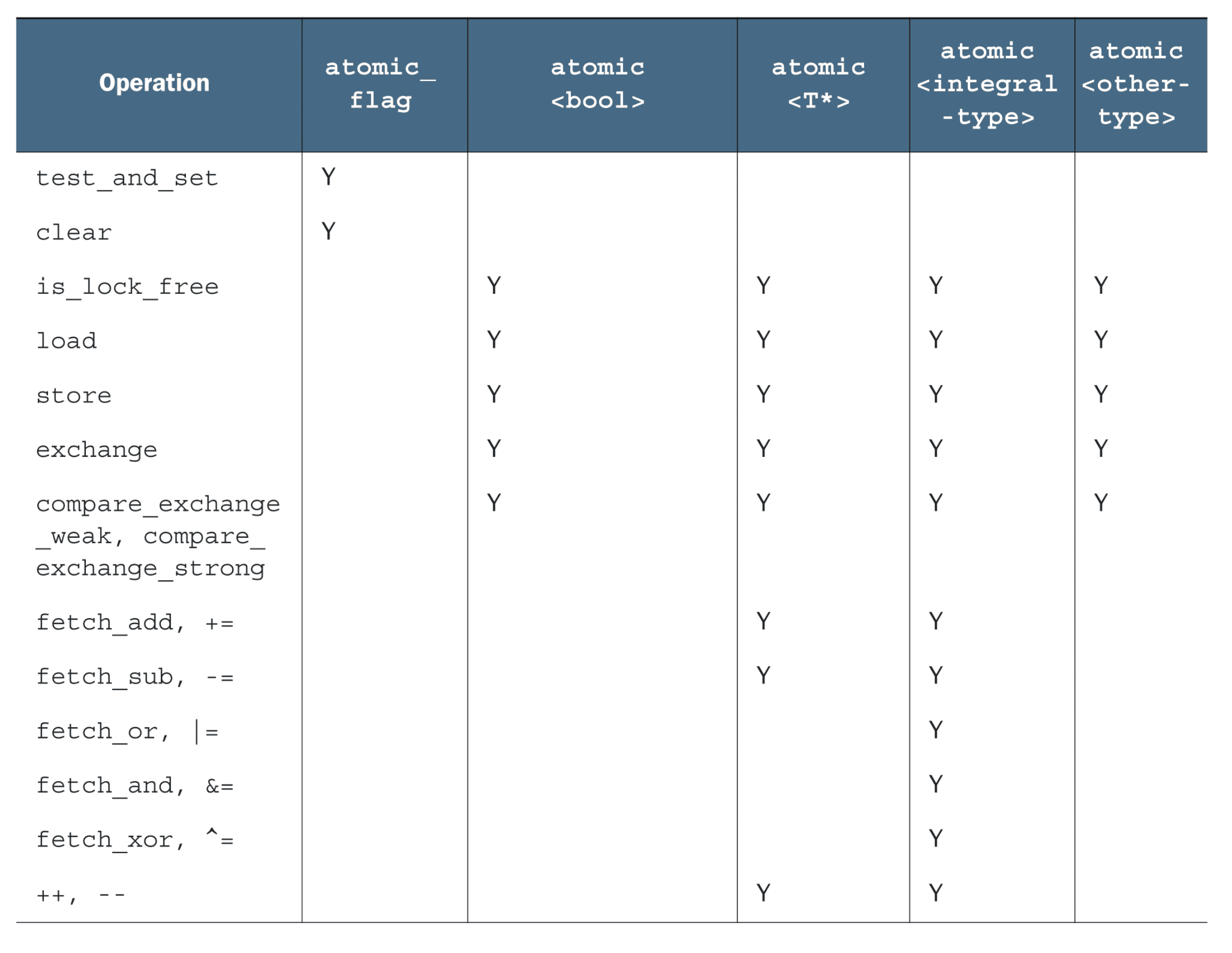# 原子操作和原子类型

## 标准原子类型

atomic_bool std::atomic<bool>
atomic_char std::atomic<char>
atomic_schar std::atomic<signed char>
atomic_uchar std::atomic<unsigned char>
atomic_int std::atomic<int>
atomic_uint std::atomic<unsigned>
atomic_short std::atomic<short>
atomic_ushort std::atomic<unsigned short>
atomic_long std::atomic<long>
atomic_ulong std::atomic<unsigned long>
atomic_llong std::atomic<long long>
atomic_ullong std::atomic<unsigned long long>
atomic_char16_t std::atomic<char16_t>
atomic_char32_t std::atomic<char32_t>
atomic_wchar_t std::atomic<wchar_t>

++

• store 操作，可选如下内存序：memory_order_relaxed、memory_order_release、memory_order_seq_cst

## std::atomic_flag

std::atomic_flag 是最简单的原子类型，这个类型的对象可以在两个状态间切换：设置和清除。就是这么简单，只作为构建块存在。正因如此，它将作为讨论其他原子类型的起点，因为它会展示原子类型可使用的策略。

std::atomic_flag 类型的对象必须被 ATOMIC_FLAG_INIT 初始化。初始化标志位是“清除”状态。这里没得选择，这个标志总是初始化为“清除”。这适用于任何对象的声明，是唯一需要以如此特殊的方式初始化的原子类型，但也是唯一保证无锁的类型。首次使用时，需要初始化。

class spinlock_mutex {
std::atomic_flag flag;
public:
spinlock_mutex(): flag(ATOMIC_FLAG_INIT) {}
void lock() {
while(flag.test_and_set(std::memory_order_acquire));
}
void unlock() {
flag.clear(std::memory_order_release);
}
};

## std::atomic

std::atomic_flag 的局限性太强，没有非修改查询操作，甚至不能像普通的布尔标志那样使用。所以，实际操作中最好使用 std::atomic。std::atomic 不保证 lock-free，可以用is_lock_free 检验在当前平台上是否 lock-free。

std::atomic<bool> b;
b.store(true);
x = b.exchange(false, std::memory_order_acq_rel);

std::atomic 提供多个“读-改-写”的操作，exchange() 只是其中之一。它还介绍了一种新的存储方式：当前值与预期值一致时，存储新值的操作。这种新型操作叫做“比较/交换”，它的形式表现为 compare_exchange_weak() 和 compare_exchange_strong()。“比较/交换”操作是原子类型编程的基石，它比较原子变量的当前值和期望值，当两值相等时，存储所提供值。当两值不等，期望值就会被更新为原子变量中的值。“比较/交换”函数值是一个 bool 变量，当返回 true 时执行存储操作，false 则更新期望值。

// 以 compare_exchange_weak 为例
// 将 a 的值与 x 比较，如果相等则把 y 赋给 a，否则把 a 赋给 x
a.compare_exchange_weak(x, y);

bool expected = false;
atomic<bool> b;
while(!b.compare_exchange_weak(expected, true) && !expected);

## std::atomic<T*>

std::atomic<T*> 为指针运算提供新的操作。基本操作有 fetch_add() 和 fetch_sub()（它们在存储地址上做原子加法和减法），以及 +=、-=、++ 和 –。举个例子，x 是std::atomic<Foo*> 类型的数组的首地址址，x.ftech_add(3) 让 x 指向第四个元素，并且函数返回指向第一个元素的地址

class Foo{};
Foo some_array;
std::atomic<Foo*> p(some_array);
Foo *x = p.fetch_add(2);  // p加2，并返回原始值
assert(x == some_array);
x = (p -= 1);  // p减1，并返回原始值
assert(x == &some_array);
assert(p.load() == &some_array);

## std::atomic<> 类模板## 原子操作的非成员函数

std::atomic<int> i(42);
int j = std::atomic_load(&i);  // i.load()

std::atomic<int> i(42);
std::atomic_load_explicit(&i, std::memory_order_acquire);  // i.load(std::memory_order_acquire)

bool compare_exchange_weak(T& expected, T desired,
std::memory_order success,
std::memory_order failure);

template<class T>
bool atomic_compare_exchange_weak(std::atomic<T>* obj,
typename std::atomic<T>::value_type* expected,
typename std::atomic<T>::value_type desired);

template<class T>
bool atomic_compare_exchange_weak_explicit(std::atomic<T>* obj,
typename std::atomic<T>::value_type* expected,
typename std::atomic<T>::value_type desired,
std::memory_order succ,
std::memory_order fail);

std::atomic_flag 对应的非成员函数的前缀不是 atomic_ 而是 _atomic_flag_，但接受 std::memory_order 参数的版本一样是 _explicit 后缀：

std::atomic_flag x =  ATOMIC_FLAG_INIT;
bool y = std::atomic_flag_test_and_set_explicit(&x, std::memory_order_acquire);
std::atomic_flag_clear_explicit(&x, std::memory_order_release);

std::shared_ptr<int> p(new int(42));
std::shared_ptr<int> q;
std::atomic_store(&q, p);

std::atomic<std::shared_ptr<int>> x; // c++20

# 原子操作的内存序

### relaxed ordering（memory_order_relaxed）

#include <atomic>
#include <assert.h>

std::atomic<bool> x,y;
std::atomic<int> z;

void write_x_then_y() {
x.store(true,std::memory_order_relaxed);  // 1
y.store(true,std::memory_order_relaxed);  // 2
}
++z;
}
int main() {
x = false;
y = false;
z = 0;
a.join();
b.join();
}

### release & acquire（memory_order_release、memory_order_acquire）

#include <assert.h>
#include <atomic>

std::atomic<bool> x, y;
std::atomic<int> z;
void write_x() { x.store(true, std::memory_order_release); }

void write_y() { y.store(true, std::memory_order_release); }

++z;
}

++z;
}
int main() {
x = false;
y = false;
z = 0;
a.join();
b.join();
c.join();
d.join();
}

#include <assert.h>
#include <atomic>

std::atomic<bool> x, y;
std::atomic<int> z;

void write_x_then_y() {
x.store(true, std::memory_order_relaxed);
y.store(true, std::memory_order_release);
}

++z;
}

int main() {
x = false;
y = false;
z = 0;
a.join();
b.join();
}

release & acquire 内存序保证同一个原子变量的 release 之前的写操作对 acquire 以后的读操作是可见的，所以说 x.load 一定发生在 x.store 之后，z 必然等于 1。

std::atomic<int> data;
std::atomic<bool> sync1(false), sync2(false);

data.store(42, std::memory_order_relaxed);
data.store(97, std::memory_order_relaxed);
data.store(17, std::memory_order_relaxed);
data.store(-141, std::memory_order_relaxed);
data.store(2003, std::memory_order_relaxed);
sync1.store(true, std::memory_order_release);  // 1.设置sync1
}

sync2.store(true, std::memory_order_release);  // 3.设置sync2
}

}

std::atomic<int> sync(0);
...
sync.store(1, std::memory_order_release);
}

int expected = 1;
while (!sync.compare_exchange_strong(expected, 2, std::memory_order_acq_rel)) {
expected = 1;
}
}

...
}

### release & consume（memory_order_release、memory_order_consume）

struct X {
int i;
std::string s;
};

std::atomic<X*> p;
std::atomic<int> a;

void create_x() {
X* x = new X;
x->i = 42;
x->s = "hello";
a.store(99, std::memory_order_relaxed);
p.store(x, std::memory_order_release);
}

void use_x() {
X* x;
assert(x->i == 42);  // 1
assert(x->s == "hello");  // 2
}

int main() {
t1.join();
t2.join();
}

memory_order_consume 很特别：完全依赖于数据。这个内存序非常特殊，即使在 C++17 中也不推荐使用。这里只为了完整的覆盖内存序而讨论，memory_order_consume 不应该出现在代码中。

### sequential consistency（memory_order_seq_cst）

#include <assert.h>
#include <atomic>

std::atomic<bool> x, y;
std::atomic<int> z;

void write_x() { x.store(true, std::memory_order_seq_cst); }

void write_y() { y.store(true, std::memory_order_seq_cst); }

++z;
}

++z;
}

int main() {
x = false;
y = false;
z = 0;
a.join();
b.join();
c.join();
d.join();
}

• 上面情况的对称
• 不管 x.store 和 y.store 谁先发生，如果 load 都发生在 store 之后，那么 z 会自增两次

# 内存栅栏

#include <assert.h>
#include <atomic>

std::atomic<bool> x, y;
std::atomic<int> z;

void write_x_then_y() {
x.store(true, std::memory_order_relaxed);             // 1
y.store(true, std::memory_order_relaxed);             // 3
}

++z;
}

int main() {
x = false;
y = false;
z = 0;
}